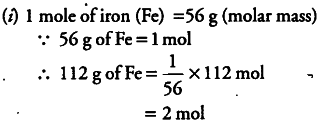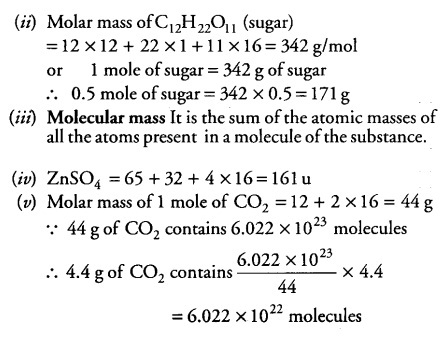# Calculate the number of moles in 112 g of iron

(i) Calculate the number of moles in 112 g of iron.
(ii) Calculate the mass of 0.5 mole of sugar
(iii) Define the term molecular mass.
(iv) Determine the molecular mass of ZnS04 [Atomic mass of Zn = 65 u, S = 32 u and
O = 16 u],
(v) Calculate the number of molecules of carbon
dioxide, C02 present in 4.4 g of C02. [Atomic mass of C = 12 u, O = 16 u and Na= 6.022 x \${10}^{23}\$ mol-1]